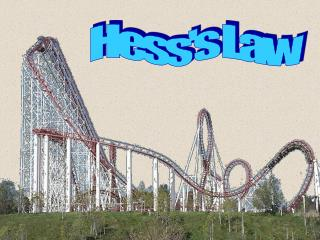Download PresentationHess's Law

Loading in 2 Seconds...

# Hess's Law - PowerPoint PPT Presentation

Hess's Law. Hess’s law. Hess’s Law states that the heat of a whole reaction is equivalent to the sum of it’s steps. For example: C + O 2  CO 2 (pg. 165) The book tells us that this can occur as 2 steps C + ½O 2  CO H = – 110.5 kJ CO + ½O 2  CO 2 H = – 283.0 kJ.I am the owner, or an agent authorized to act on behalf of the owner, of the copyrighted work described.
Download Presentation## Hess's Law

An Image/Link below is provided (as is) to download presentation

Download Policy: Content on the Website is provided to you AS IS for your information and personal use and may not be sold / licensed / shared on other websites without getting consent from its author.While downloading, if for some reason you are not able to download a presentation, the publisher may have deleted the file from their server.

- - - - - - - - - - - - - - - - - - - - - - - - - - E N D - - - - - - - - - - - - - - - - - - - - - - - - - -
Presentation Transcript
1. Hess's Law

2. Hess’s law • Hess’s Law states that the heat of a whole reaction is equivalent to the sum of it’s steps. • For example: C + O2 CO2 (pg. 165) The book tells us that this can occur as 2 steps C + ½O2  CO H = – 110.5 kJ CO + ½O2  CO2 H = – 283.0 kJ C + CO + O2  CO + CO2 H = – 393.5 kJ I.e. C + O2  CO2 H = – 393.5 kJ • Hess’s law allows us to add equations. • We add all reactants, products, & H values. • We can also show how these steps add together via an “enthalpy diagram” …

3. Steps in drawing enthalpy diagrams • Balance the equation(s). • Sketch a rough draft based on H values. • Draw the overall chemical reaction as an enthalpy diagram (with the reactants on one line, and the products on the other line). • Draw a reaction representing the intermediate step by placing the relevant reactants on a line. • Check arrows: Start: two leading away Finish: two pointing to finish Intermediate: one to, one away • Look at equations to help complete balancing (all levels must have the same # of all atoms). • Add axes and H values.

4. C + O2  CO2 H = – 393.5 kJ C + O2 C + ½O2  CO H = – 110.5 kJ CO + ½O2  CO2 H = – 283.0 kJ Reactants H = – 110.5 kJ CO + ½O2 Intermediate Enthalpy H = – 393.5 kJ H = – 283.0 kJ CO2 Products Note: states such as (s) and (g) have been ignored to reduce clutter on these slides. You should include these in your work.

5. Practice Exercise 6 (pg. 167) with Diagram C2H4(g) + 3O2(g) 2CO2(g) + 2H2O(l) H= –1411.1 kJ 2CO2(g) + 3H2O(l)  C2H5OH(l) + 3O2(g) H= +1367.1 kJ Using example 5.6 as a model, try PE 6. Draw the related enthalpy diagram. C2H4(g) + H2O(l)  C2H5OH(l) H= – 44.0 kJ C2H4(g) + H2O(l) + 3O2(g) Reactants Products C2H5OH(l) + 3O2(g) H= –44.0 kJ H = –1411.1 kJ H = +1367.1 kJ Enthalpy 2CO2(g) + 3H2O(l) Intermediate

6. 5.51 (pg. 175) GeO(s)  Ge(s) + ½ O2(g) H= + 255 kJ Ge(s) + O2(g)  GeO2(s) H= – 534.7 kJ GeO(s) + ½ O2(g)  GeO2(s) H= – 279.7 kJ Ge(s) + O2(g) Intermediate H = +255 kJ GeO(s) + ½ O2(g) H = – 534.7 kJ Reactants Enthalpy H= –280 kJ GeO2(s) Products

7. 5.52 (pg. 175) NO(g)  ½ N2(g) + ½ O2(g) H= – 90.37 kJ ½ N2(g) + O2(g)  NO2(g) H= + 33.8 kJ H= – 56.57 kJ NO(g) + ½ O2(g)  NO2(g) NO + ½ O2(g) Reactants H= –56.6 kJ NO2(g) H = –90.37 kJ Enthalpy Products H = +33.8 kJ ½ N2(g) + O2(g) Intermediate

8. Hess’s law: Example 5.7 (pg. 166) We may need to manipulate equations further: 2Fe + 1.5O2  Fe2O3 H=?, given Fe2O3 + 3CO  2Fe + 3CO2 H= – 26.74 kJ CO + ½O2  CO2 H= –282.96 kJ 1: Align equations based on reactants/products. 2: Multiply based on final reaction. 3: Add equations. 2Fe + 3CO2  Fe2O3 + 3CO H= + 26.74 kJ CO + ½O2  CO2 H= –282.96 kJ 3CO + 1.5O2  3CO2 H= –848.88 kJ 2Fe + 1.5O2  Fe2O3 H= –822.14 kJ Don’t forget to add states. Try 5.55, 5.57, 5.58, 5.61 (pg. 175) For more lessons, visit www.chalkbored.com